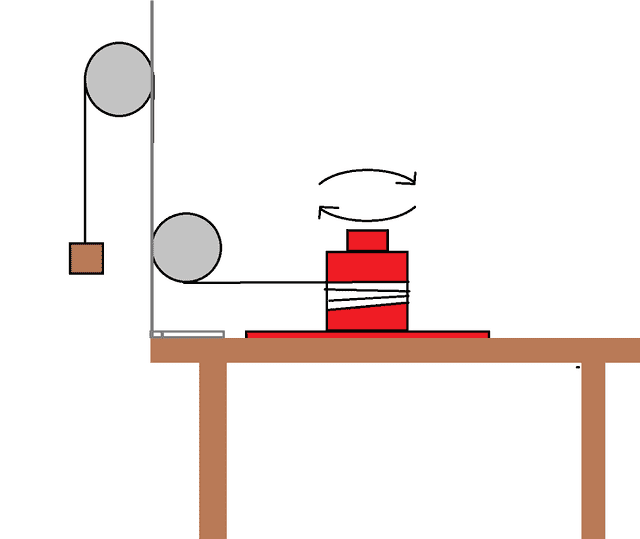# Energy and Rotational Motion

## Homework Statement

We performed a small experiment in class which had us attach a mass to a string which hung on a pulley which led to a rotating object.We were then told to write down a conservation of energy equation stating that the initial energy is equal to the final energy. We were asked to include kinetic energy (rotational, translational), potential energy, and energy lost due to friction which we found with Tf=f*r.

## The Attempt at a Solution

This is what I have setup so far.

Ui +Kroti +Ktransi=Uf+Krotf +Ktransf +Wf

mgd=.5Iw^2 + .5mv^2 -f

I'm still a little confused about how energy is conserved if we're losing energy due to friction?

After that they ask me to replace every instance of w with v/r and v with 2d/t and try to get the following I=(mr^2)((gt^2/2d)-1-(t^2/(2md))f)

What am I doing wrong here?

## Answers and Replies

haruspex
Science Advisor
Homework Helper
Gold Member
2020 Award
mgd=.5Iw^2 + .5mv^2 -f
f is the force of friction? If so, there are two things wrong with the -f term.

how in the world are you equating torque with energy

f is the force of friction? If so, there are two things wrong with the -f term.
I suppose I should have did f*Δd? I'm not sure what to do here.